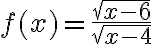Difficulty Finding the Domain of a Function using Interval Notation.

denniswood

New member
Hi everyone, I'm having difficulty figuring out how to answer these two questions below, specifically the first one. I have been trying for days to answer this question but can't figure it out. If anyone could help me out on these two answers, I would be so grateful.

1. Find the domain of the function using interval notation.2. Sketch a graph of a piecewise function. Write the domain in interval notation.

y = x^2 {-1 < x < 1}
and
y = 3x - 2 {1 < x < 3}

Last edited by a moderator:

Harry_the_cat

Senior Member
Ok to help with Q1, can you first answer these questions:
1. For $$\displaystyle \frac{1}{x}$$ to be defined, what CAN'T x be?
2. For $$\displaystyle \sqrt{x}$$ to be defined what CAN'T x be?

•Otis

denniswood

New member
Ok to help with Q1, can you first answer these questions:
1. For $$\displaystyle \frac{1}{x}$$ to be defined, what CAN'T x be?
2. For $$\displaystyle \sqrt{x}$$ to be defined what CAN'T x be?
This is why I am having such difficulty because those aspects of what x CAN'T be were NOT listed in the assignment question, for either of the questions, which is why I have been unable to identify what it is I must do.

Otis

Senior Member
… having difficulty figuring out how to answer these …

… Sketch a graph of a piecewise function. Write the domain in interval notation …
Hello. I'm not sure where you're having difficulty sketching. Do you know how to sketch y=x^2 by itself? Can you sketch the line y=3x-2?

Also, the two intervals (that is, the two sets of numbers comprising the domain) have been given to you. Is 'interval notation' the difficulty? Or, maybe you haven't learned the definition of 'domain', yet.

Please follow the forum's submission guidelines, and be specific. Show your efforts, thus far. Thanks!Last edited:

Otis

Senior Member
… I am having such difficulty because those aspects of what x CAN'T be were NOT listed in the assignment question …
Maybe that information wasn't provided because you're expected to have already learned it in pre-algebra.

We cannot divide by zero, so the expression 1/x is defined for all Real numbers x except 0. In other words, the denominator of the algebraic fraction in your first exercise cannot be zero.

√(x - 4) will be zero IF x - 4 = 0, and we cannot allow that to happen, so what value of x causes the denominator to become zero?

Next, we can't take the square root of negative numbers, so √x is defined only when x≥0. In other words, in the numerator the radicand cannot be negative. (The radicand is what we call the expression inside a radical sign.)

The radicand in the numerator is x - 6. It can't be negative. So, solve the inequality x-6≥0.

Please show your work, up to this point, and we can go from there.

By the way, you posted on the Advanced Math board, but this is high-school level math (and pre-algebra was 8th-grade math, at my school). What class are you taking?JeffM

Elite Member
This is why I am having such difficulty because those aspects of what x CAN'T be were NOT listed in the assignment question, for either of the questions, which is why I have been unable to identify what it is I must do.
The domain of a real function is the set for which the function is defined to be a real number. In many cases, a domain is not explicitly specified. In those cases, we want or need to know the maximum possible domain. The usual way to do that is to exclude what cannot possibly be in the domain. More concretely, the maximum possible domain is all real numbers except those where where the function will not be a real number.

You have a function in the form $$\displaystyle f(x) = \dfrac{\sqrt{u}}{v} \text { and } v = \sqrt{w}.$$

So where can't the function be a real number?

Well clearly v = 0 is excluded, and v will equal 0 only if w = 0. Also clearly excluded are w < 0 and u < 0. But these are all functions of x. Putting them all together what values of x are excluded? Then the maximum oossible domain is everything else.

Personally, I think these questions are badly worded. Instead of asking "what is the domain," what should be asked is "what is the maximum possible domain." In that case, it becomes obvious that the way to answer is to determine what cannot be in the domain and include everything else.